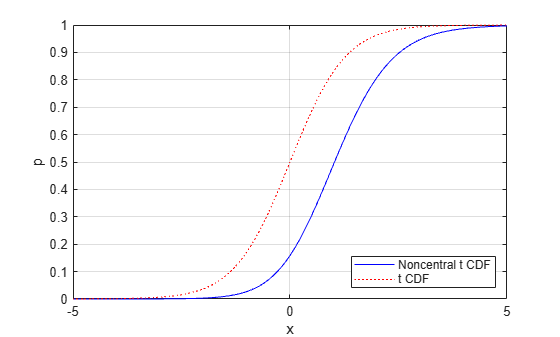# nctcdf

Noncentral t cumulative distribution function

## Syntax

```p = nctcdf(x,nu,delta) p = nctcdf(x,nu,delta,'upper') ```

## Description

`p = nctcdf(x,nu,delta)` computes the noncentral t cdf at each value in `x` using the corresponding degrees of freedom in `nu` and noncentrality parameters in `delta`. `x`, `nu`, and `delta` can be vectors, matrices, or multidimensional arrays that have the same size, which is also the size of `p`. A scalar input for `x`, `nu`, or `delta` is expanded to a constant array with the same dimensions as the other inputs.

`p = nctcdf(x,nu,delta,'upper')` returns the complement of the noncentral t cdf at each value in `x`, using an algorithm that more accurately computes the extreme upper tail probabilities.

## Examples

collapse all

Compare the noncentral t cdf with `DELTA` = `1` to the t cdf with the same number of degrees of freedom (10).

```x = (-5:0.1:5)'; p1 = nctcdf(x,10,1); p = tcdf(x,10); plot(x,p,'-',x,p1,':')```## References

 Evans, M., N. Hastings, and B. Peacock. Statistical Distributions. 2nd ed., Hoboken, NJ: John Wiley & Sons, Inc., 1993, pp. 147–148.

 Johnson, N., and S. Kotz. Distributions in Statistics: Continuous Univariate Distributions-2. Hoboken, NJ: John Wiley & Sons, Inc., 1970, pp. 201–219.

## Version History

Introduced before R2006a